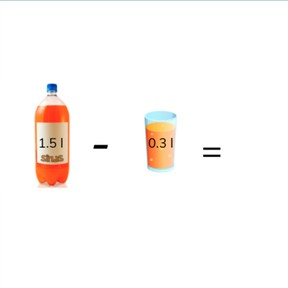Subtraction with decimal numbers with 1 and 2 decimal places

# Subtraction with decimal numbers with 1 and 2 decimal places8,000 schools use Gynzy92,000 teachers use Gynzy1,600,000 students use Gynzy

## General

Students learn to subtract decimal numbers with 1 and 2 decimal places. They also learn to subtract decimal numbers in story problems.

5.NBT.B.7

## Relevance

It is important that students can subtract decimal numbers with 1 and 2 decimal places, so they can determine for example, how far they still need to walk on a hike.

## Introduction

Students practice subtraction problems with decimal numbers with 1 decimal place and with 2 decimal places.

## Development

Using the number line, explain how you can subtract decimal numbers with 1 and 2 decimal places from each other. Show two methods to solve such subtraction problems. First, show how to take away 87.6 from 42.33. You split the 42.33 into 42 and 0.33. Using the number line, take away 42. Then take away the 0.33. Next show students the second method. Show students that you split both the first and second numbers into whole numbers and decimal numbers. In this case 87.6 - 42.33 becomes 87 - 42 and 0.6 - 0.33. Solve both subtraction problems and add them together to find the difference. Explain to students that this method only works if the first decimal number is greater than the second decimal number. Use the next problems to check that students are able to subtract decimal numbers with 1 and 2 decimal places. Make sure to repeat that students can choose the method they like best or find easiest to use.
Next discuss the story problem. Show students the steps required to solve a story problem. First determine what kind of problem it is, then determine which numbers you need for the problem. Then say or write the problem, and finally, solve the problem. Solve a story problem as a class and then ask students to solve the next individually or in pairs. Remind students to name the steps they take to solve the problem.

Check that students are able to subtract decimal numbers with 1 and 2 decimal places by asking the following questions:
- How can you solve subtraction problems with 1 and 2 decimal places?
- Which method do you like best? Why?
- How do you solve a story problem?
- What are the steps to solve a story problem?

## Guided practice

Students are given subtraction problems with decimal numbers with 1 and 2 decimal places. They are asked to fill in the answer or to select from multiple choice answers. They are also asked to solve decimal number story problems, where they must fill in the answer or select from multiple choice answers.

## Closing

Check that students are able to explain how they subtract decimal numbers with 1 and 2 decimal places . Check that they are able to name the steps in solving a story problem. Use the problems on the interactive whiteboard to check that students are comfortable solving these problems individually. Repeat that students may select the method to use to find the difference. Have students form pairs to solve the last subtraction problems with receipts. Have them determine how much money they have left after paying for the indicated items.

## Teaching tips

Students who have difficulty subtracting decimal numbers with 1 and 2 decimal places can be supported by the use of a number line. Have them practice subtracting in steps of 0.1 and 0.01 from a given number. Make sure to also cover the transition from 1 to 0.9, from 10 to 9.9 as well as 1 to 0.99 and 10 to 9.99.

## Instruction materials

Optional: Number line

### The online teaching platform for interactive whiteboards and displays in schools

• Save time building lessons

• Manage the classroom more efficiently

• Increase student engagement